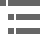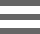## Geometrical Construction of Ray DiagramsA popular method of representing a train of propagating light waves involves the application of geometrical optics to determine the size and location of images formed by a lens or multi-lens system. This tutorial explores how two representative light rays can establish the parameters of an imaging scenario.

Traces of three component light rays belonging to an oblique wave passing through a perfect, aberration-free lens are presented in the tutorial. The height (and size) of the object (h') and image (h) are controlled by the Object Height slider, which can be utilized to adjust the relative height of the object and image. Adjusting the object height will produce minute fluctuations in the image magnification due to limitations of the algorithms utilized in construction of the tutorial. Focal point positions can be altered by translating the Focal Length slider to the left (shorter focal lengths) or right (longer focal lengths). Moving this slider simultaneously changes the position of both the front (F') and rear (F) focal points, which lie on the optical axis of the lens system in the object space and image space, respectively. Image magnification is also a function of focal point location.

The positions of the object and image, with respect to the lens and focal points, are controlled by the Object Position slider, which simultaneously invokes changes in magnification. Principal planes passing through the center of the lens can be translated through a small range along the optical axis with the Principal Planes slider. Important relationships, including the lens makers' equation (see below) and the lateral magnification are calculated and displayed in the tutorial window as control parameters are adjusted.

Two representative light rays, one paraxial and one traveling through the center of the lens (the principal ray), are employed to establish the parameters of the imaging situation. Many textbooks on Gaussian optics refer to these light rays as characteristic rays, with the principal ray being the one that passes through the center of the entrance and exit pupils, the lens, and any aperture diaphragms present in the optical system. Often, the principal ray is omitted from consideration and the characteristic rays passing through the front and rear focal points of the lens are utilized to define the object and image size and location. In the tutorial, the second characteristic ray is illustrated as ray trace line passing through the front focal point (F') of the lens.

The object is located at distance a to the left of the lens, in the region known as the object space. A single light ray, designated by the line emanating from the top of the object and intersecting the optical axis at the object-side focal point (F'), is refracted by both surfaces of the lens and exits parallel to the optical axis. Extensions of the refracted and incident ray intersect at a surface within the lens located at distance a from the object. This surface is known as the first or object-side principal surface, and is designated by P' in the tutorial. The uppermost light ray, progressing from the object in a direction parallel to the optical axis, is refracted by the lens and passes through the image-side focal point (F). Extensions of the refracted and incident ray intersect at the image-side principal surface (noted as P in the tutorial window) within the lens at distance b from the image. Near the vicinity of the lens axis, the surfaces P' and P approximate plane surfaces and are known as the principal planes of the lens. The intersections of these planes with the optical axis of the lens are referred to as the principal points.

Another set of points utilized to define lens parameters are the nodal points, which occur where the extensions of oblique light rays passing through the lens intersect the optical axis. Nodal points are not illustrated in the tutorial, but would lie very close to the principal points of the lens. Thus, three pairs of points, the lens focal points (F and F'), principal planes (P'and P), and the principal nodes all lie on the lens optical axis. If the location of the focal points and either the principal points or the nodal points is known, then geometrical construction of ray traces to elucidate object and image parameters can be undertaken without considering refraction of light rays at each surface of the lens. The result is that any lens system can be simulated utilizing only the focal points and principal planes by drawing ray traces as if they encounter the first principal plane, travel parallel to the optical axis, and emerge from the second principal plane without refraction.

Note that distance a is greater than the lens front focal length, F' in the tutorial. Under these circumstances, an inverted image is then formed in the image space at a distance b to the right of the lens. The length of b is greater than the lens rear focal length, F, which is related to the distances a and b by the equation:

The image height is denoted by the quantity h, and represents an increase in size resulting from magnification of the object or specimen positioned at the front of the lens and having a height of h'. The lateral magnification, M of this simple lens (which approximates a Gaussian thin lens) is expressed by the equation:

Because the object and image lie in conjugate planes, points in the image will be focused at corresponding points on the object by the lens. The focal length would then be represented by F' and the magnification (M) inverted to 1/M due to the reduction in size of the image when the reverse situation is considered.

The distance ratio between two image points along the optical axis on the object side of the lens and two conjugate points on the image side is known as the longitudinal or axial magnification. The magnitude of the longitudinal magnification is the square of the lateral magnification for small distances from the image plane. All of the imaging components in the optical microscope are governed by the basic geometrical relationships described above. This includes the collector lens, condenser, objective, eyepieces (in the projection mode), camera system, and the human eye.

### Contributing Authors

Matthew Parry-Hill and Michael W. Davidson - National High Magnetic Field Laboratory, 1800 East Paul Dirac Dr., The Florida State University, Tallahassee, Florida, 32310.

# Microscopy U - The source for microscopy education•••